# Magic Rectangle

## Sparking Curiosity From Multiplication to Quadratic Relationships

In this 3 act math task, students watch a rectangle that is iterating between getting “shorter” and “wider”, then “taller” and “thinner”.

While this task might seem simple on the surface, there is a lot of “mathemagic” going on in the background.

Jon Orr and I planned this task while driving home from a conference back in November 2017 and we are now only finding the time to get it online due to so many requests for sharing from the recent workshops we’ve used it in. The initial intention was to create a problem that would unpack some of the important details of quadratic equations including creating equations and manipulating between factored, standard, and vertex form as well as developing a need for the use of completing the square concretely with algebra tiles. However, this task can be accessible by students in grade 5 when working with two-digit multiplication and area, while also having the opportunity to extend all the way to optimization problems in calculus.

In particular, this task can be used for building conceptual understanding as well as procedural fluency in:

• estimation;
• spatial reasoning;
• two-digit by two-digit multiplication;
• area of rectangles;
• tables of values and graphs of non-linear relationships;
• creating quadratic equations in factored, standard, and vertex form;
• completing the square with concrete materials (algebra tiles);
• optimization problems in calculus; and,
• many more.

Let’s get started!

## Act 1: Sparking Curiosity

Ask students to create a chart that says “notice” and “wonder” at the top.

While they watch the following video clip, have them independently jot down everything they notice and wonder.

Give your students some time to share out what they noticed and wondered while watching the video with their neighbours. I usually give about 90 seconds for this.

Then, share out to the entire class.

Here are some examples of things they may have noticed or wondered:

• A rectangle.
• A square at the start.
• Is the perimeter the same the whole time?
• When is the rectangle covering the most area?
• Why are we doing this?
• And many others…

After taking the time to jot down each notice and wonder, as well as respecting each as equally as possible (i.e.: not getting super excited for one and not another), we will try to narrow down to a question such as:

When is the area the greatest?

Give students an opportunity to make an estimate by first thinking independently and then having them share their prediction with a neighbour.

Replaying the first video is something I typically do at this point.

Then, I slowly move through specific rectangles by progressing through each one slide by slide.

The animated gif below gives you the effect: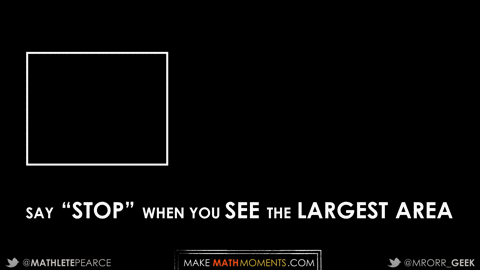Let your students update their estimates with their neighbours and then I’ll take estimates from the class and write them up on the board.

At this point, I will typically have them go to the whiteboards / chalkboards set up around my room to engage in a vertical non-permanent surface (VNPS) experience to solve the problem.

Don’t have any whiteboards or chalkboards in your classroom? The great folks at Wipebook are offering Educator Starter Packs for the Tap Into Teen Minds / Make Math Moments learning community for 25% off using this link for Canadian addresses and this link for US/Outside Canada. I use Wipebook flip charts for when I’m presenting in rooms without vertical non-permanent surfaces to maximize engagement and learning!

Full disclosure: Wipebook is flipping you 25% off and they are flipping Jon Orr and I a small percentage of their profit to help support our mission to bring you the best math activities and resources! Win-win!

## Act 2: Reveal Information to Fuel Sense Making

Now that students are at the Vertical Non-Permanent Surfaces, I’ll begin revealing some information.

Here’s what I typically share: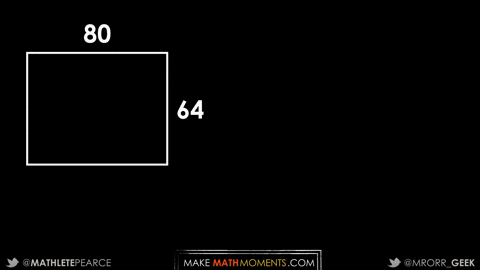At this point, most students are off to the races.

Depending on the class I’m in, I anticipate seeing some of these strategies with the grades/courses:

• a bunch of rectangles with dimensions and the area drawn repeatedly (Grade 5 – Calculus);
• a table of values (Grade 7 – Calculus);
• graphs (Grade 9 – Calculus);
• completing the square (Grade 10 – Calculus, although I’m very surprised if I see this);
• optimization strategies using derivatives (Calculus); and,
• many others in between!

As mentioned earlier, I created this task as a great way to spark curiosity to fuel sense making and essentially help students see the value in completing the square in grade 10 academic (and beyond). Completing the square was always a topic that was too abstract for me as a student and I actually didn’t really understand how it worked when I was teaching Grade 10, either. Now that I feel more confident in this area, I wanted to create a task to intentionally address this topic.

When working with high math teachers recently, here’s where they went after having the dimensions of the first 4 rectangles.

1) Area of Rectangle Diagrammatically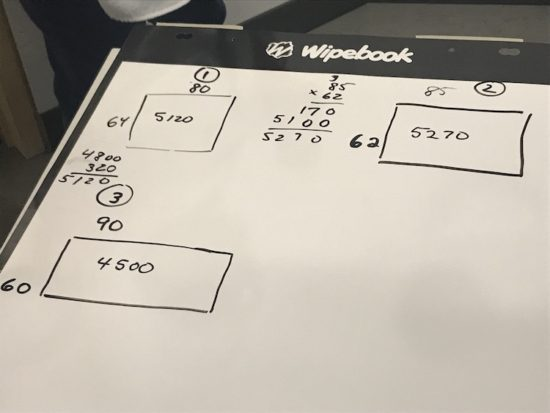2) Area of Rectangle Diagrammatically and Table of Values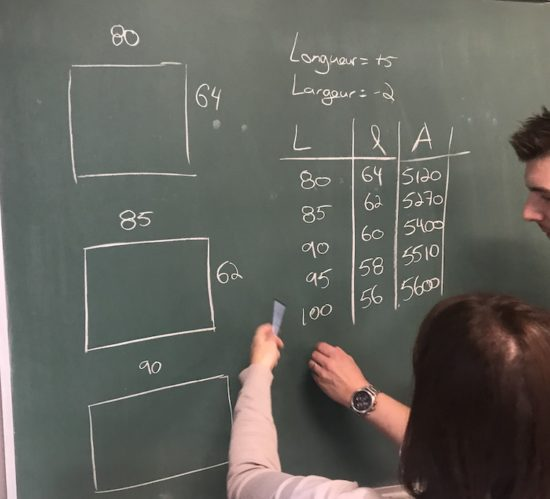3) Table of Values and Graph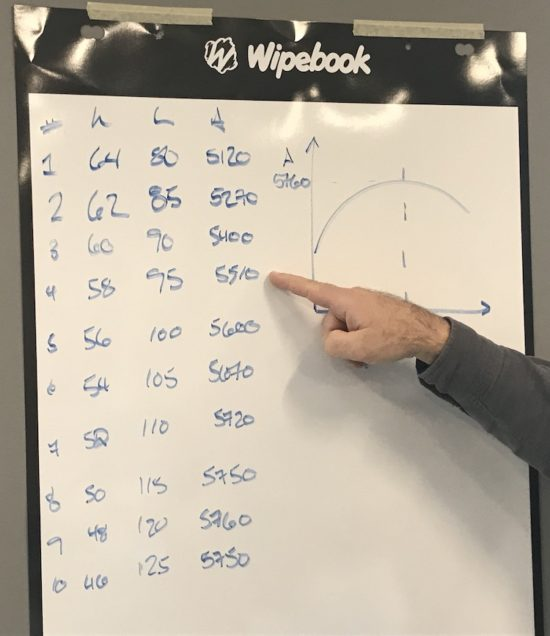4) Area of Rectangle Diagrammatically, Quadratic Equation in Factored Form, Expanded to Standard Form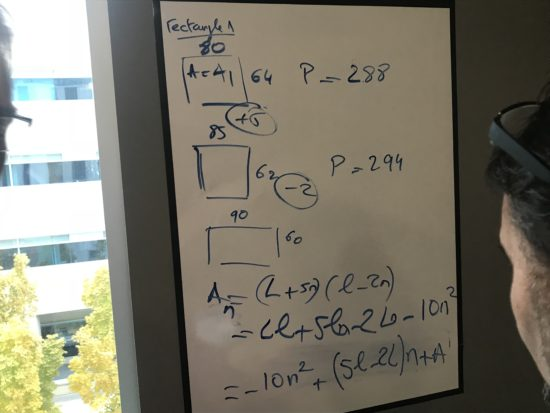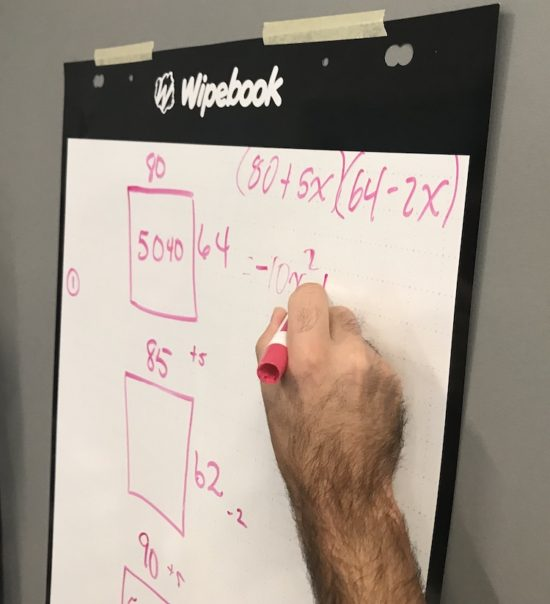5) Area of Rectangle Diagrammatically, Quadratic Equation in Factored Form, Then Completing the Square to Find Vertex Form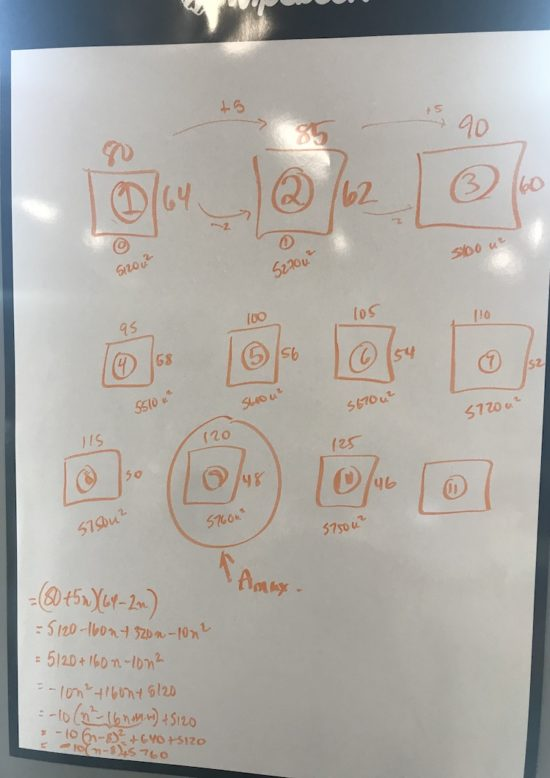I completely understand that these are solution strategies shared by high school teachers and thus results will vary significantly in the classroom. However, I’d argue that since we’ve withheld so much information and i haven’t revealed a specific learning goal (yet), even teachers are forced to really dig into their prior knowledge to pull together a problem solving strategy without solely relying on a procedure that they’ve memorized for specific recognizable situations.

Although unnecessary, you might choose to show the following animated gif if you feel it is helpful: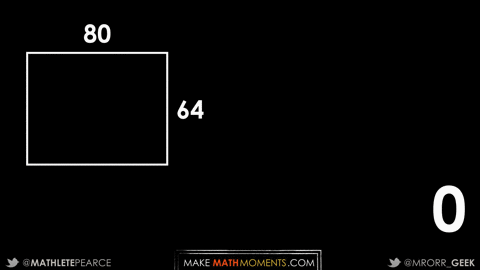## Fuel Sense Making: Consolidating the Learning

Once students have been given enough information to now make calculations to improve their predictions, we will use the 5 Practices for Orchestrating Productive Mathematics Discussions to Fuel Sense Making and Consolidate the learning. While we should already be anticipating prior to the lesson to prepare ourselves for what we might see during this stage of the lesson, we are now Monitoring, Selecting, Sequencing and finally Connecting student work to the learning goal for the day.

To paint a picture of what this looks like in action, I’m walking around the room monitoring the work of each group working at their vertical non-permanent surfaces, asking questions to better understand their thinking and to help press them for understanding.

If they have shown a bunch of rectangles with the area calculated, I might ask how they can organize their work (i.e.: pushing for a table of values).

If they have a table of values, I might ask them what they think the graph would look like and why? After they share, I ask them to prove it (i.e.: pushing them to actually create a graph).

If they have a table of values and/or a graph, I might ask them to describe the relationship for ANY term (i.e.: the nth term or the general equation). Sometimes it can be helpful for students to go back to the diagrammatic or visual of multiple rectangles and their areas because it is often easier to “see” factored form of a quadratic equation from that point. For example, the first rectangle is 80 by 64 with the length increasing by 5 each time and the width decreasing by 2 each time. This can make it easier for students to see y = (80 + 5x)(64 – 2x).

From here, many students often can expand to standard form, but that doesn’t yield too much useful information.

At this point, all students are able to access this task and can determine the rectangle with the maximum area via area diagrammatically, from the table of values or from the graph. Each of those methods are effective, but wouldn’t it be great to have a more efficient method to save a bit of time and effort?

Boom. Enter completing the square.

We now have a reason to teach this method. The hard part is helping students understand how it works conceptually.

So let’s get to it!

While I’d prefer to bring students around the work of their peers who have found factored form, I have some visuals prepared in the case that no groups have used that strategy.

Here’s what it might look like: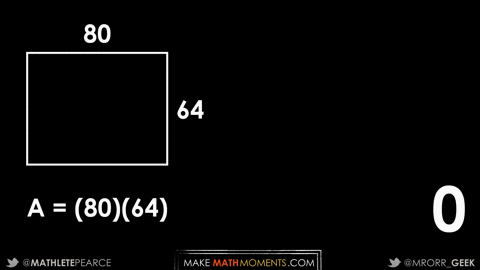If students have created graphs, we can use the x value of the vertex to substitute into factored form and confirm what the maximum area is. Here, I’ve used screenshots from Desmos free online graphing calculator: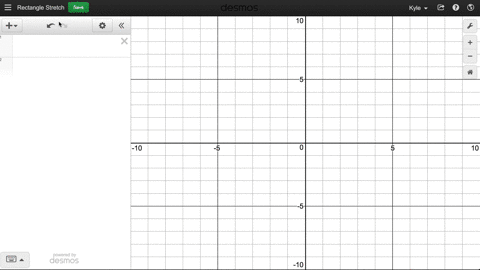Students could also use factored form to find the zeros or roots of the quadratic relationship:

y = (80 + 5x)(64 – 2x)

If we let y = 0, we can solve for the roots:

0 = 80 + 5x
-5x = 80
x = -16

and

0 = 64 – 2x
2x = 64
x = 32

If we find the midpoint between -16 and 32, we find the x-value of the vertex: x = 8.

Although some students may show the expansion of factored form to standard form, if students all did so algebraically, I might want to ensure that other students are aware of using partial products just like we can use for multiplication and the standard algorithm for multiplication: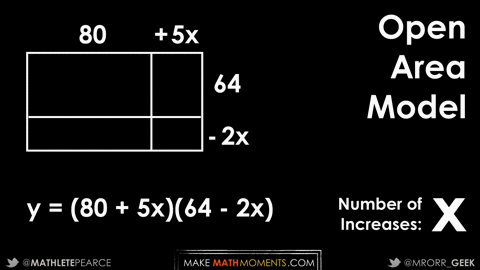Finally, another connection – the big connection Jon and I created this task for – was representing the quadratic equation in vertex form by completing the square.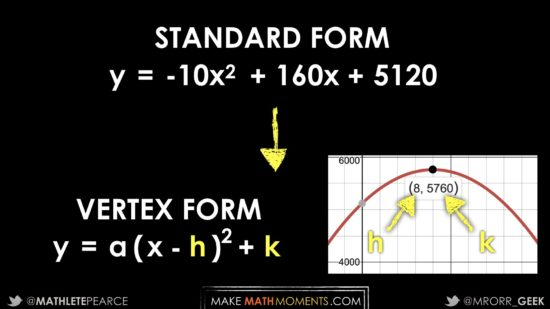Just a handful of years ago, teaching students how to complete the square would be strictly procedural in nature. However, I now realize that there is a better way. A much more concrete and visual way.

Let’s start with a simple trinomial, say y = x^2 + 4x + 5 and let’s look at it visually.

Since vertex form is y = a(x – h)^2 + k, we need to complete a (x – h) “square”.

Here’s what it looks like: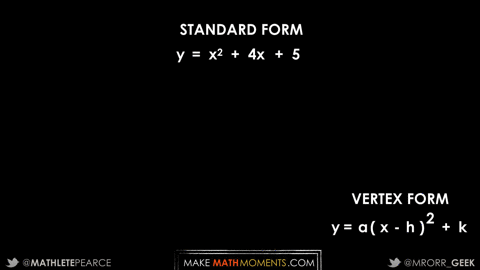You’re basically just taking the pieces of your trinomial and trying to make a square. Your leftover pieces (or missing pieces, if negative) are your value of k; the y-value of the vertex.

So when we look at our quadratic of interest in standard form, we are dealing with y = -10x^2 + 160x + 5120.

Wow, that’s not pretty.

However, if we keep in mind that because we have 10 negative x^2 pieces to work with, that means we are building -10 “copies” of the same square.

So, let’s just focus on one copy. We can do so by common factoring out the -10 from each term and focus on one square that is scaled (down) by a factor of -1/10.

Here’s what this looks like: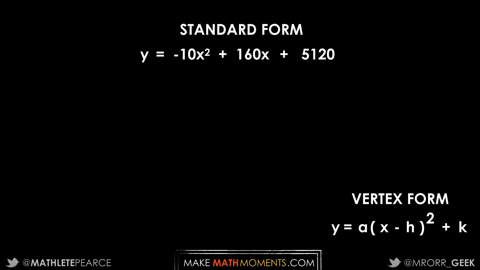## Act 3: Reveal the Answer

Act 3 was really much earlier when students all gain the realization of which rectangle is the “magic rectangle” with the greatest area.

However, just to seal the deal, the Magic Rectangle is: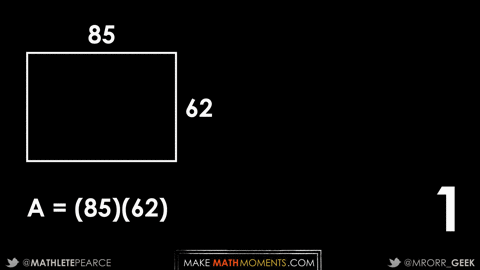Download the videos, animated gifs, and other resources to make sure that the Magic Rectangle 3 Act Math Task so you can spark curiosity to fuel sense making in your classroom!## How’d It Go?

Both Jon and I are super eager to hear about how this task goes in your classroom. We’d love to see student solutions and your feedback on social media and in the comments below. How does this task spark curiosity and fuel sense making in your classroom?

Looking forward to hearing from you!

## New to Using 3 Act Math Tasks?

Download the 2-page printable 3 Act Math Tip Sheet to ensure that you have the best start to your journey using 3 Act math Tasks to spark curiosity and fuel sense making in your math classroom!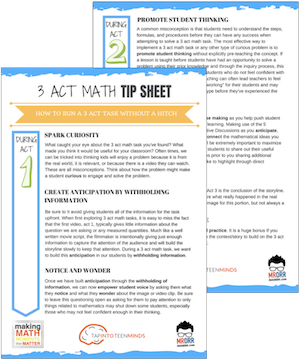## Share With Your Learning Community:I’m Kyle Pearce and I am a former high school math teacher. I’m now the K-12 Mathematics Consultant with the Greater Essex County District School Board, where I uncover creative ways to spark curiosity and fuel sense making in mathematics. Read more.

Access Other Real World Math Tasks

## Search More 3 Act Math Tasks

Spark Curiosity!